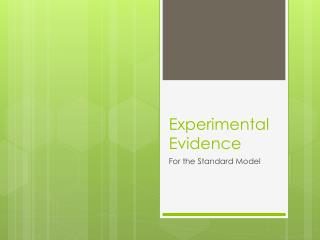Download PresentationExperimental Evidence

# Experimental Evidence

Download Presentation## Experimental Evidence

- - - - - - - - - - - - - - - - - - - - - - - - - - - E N D - - - - - - - - - - - - - - - - - - - - - - - - - - -
##### Presentation Transcript

1. Experimental Evidence For the Standard Model

2. The Ω- particle As we have learned (and done), Gell-Mann categorized by spin. The spin-3/2 baryons were then sorted by charge and strangeness. The geometry showed him (and you) that there was a missing, yet to be discovered particle. This was indirect evidence for quarks. The direct evidence appeared from deep-inelastic scattering.

3. Deep-Inelastic Scattering In a deep-inelastic scattering experiment, a lepton (like an electron) scatters off a hadron (like a proton)and transfers large amounts of energy and momentum to the hadron. The term ‘inelastic’ refers to the fact that after the collision, new hadrons are produced. The basic idea behind this experiment is that the leptons behave differently when they scatter off a particle with no structure then a particle which has one.

4. Deep Inelastic Scattering It is found that the scattering angles an energies of the leptons are consistent with the existence of very small, hard objects inside the proton. These experiments can measure the probability that a given consistuent carries off a fraction of the original momentum. The fraction of momentum carried off is given the label, x.

5. Deep Inelastic Scattering The most probable outcome is that this consistuent carries away roughly 1/3 of the total momentum. This is consistent with the expectation that, with three quarks in the proton, each would carry, on average, 1/3 of the momentum. Conclusion #1: There are 3 small constituent particles inside baryons and 2 inside mesons. This is evidence for quarks.

6. Deep Inelastic Scattering The electron and the proton exchange energy and momentum through the virtual photon. This is an electromagnetic interaction and therefore should have a strength of αEM. (This value is based on the charge.) However, the strength is a bit smaller and the experiment shows that the quarks charge is ±1/3 or ±2/3 Conclusion #2: The consistuent particles have fractional charge.

7. Deep Inelastic Scattering The amplitude of the vertex showing an electron scattering off a quark has an amplitude of A. These experiments show that the amplitude is 3A and this means there are 3 Feynman diagrams for the same interaction. This is evidence for color charge. Conclusion #3: Each of the consistuent particles appears to come in three types.

8. Deep Inelastic Scattering Conclusion 4: There appear to be electrically neutral constituents inside hadrons This is evidence for gluons. Because the interaction is electromagnetic, the electron can ‘see’ only charged constituents of the proton. In these experiments, we can measure the momentum of the particles to which the electron couples. It is found that the total momentum of these particles is less than that of the proton itself. We deduce that there are other constituents inside the proton that are neutral (gluons).

9. Deep Inelastic Scattering Conclusion 5: The particles inside the hadron behave essentially as free particles (they are loosely bound to each other). Just like a ball hitting a group of marbles would react differently than a ball hitting marbles glued together, the electron scattering off an individual quark bounces off differently then if it hit bound quarks. This is evidence for asymptotic freedom.

10. Asymptotic Freedom The strength of the interactions are approximately: αEM = 1/137 αW = 10-6 αS = 1 The fact that αs =1 poses serious problems We saw that the vertex of any Feynman diagram is assigned a factor of α0.5. This means that is α<1, diagrams with many vertices are less likely to occur. Therefore it is a safe approximation to consider the simplest Feynman diagram (with one vertex).

11. Asymptotic Freedom But for the strong interaction, αS=1, so all Feynman diagrams are equally likely to occur. αS x αS x αs x … = 1 This means there would be too many diagrams to consider. However, in the 1970s, it was realized that these interaction strengths depend on the energy transferred at the vertices. It was shown that the strong interaction strength αS actually decreased as the energy increased.

12. Asymptotic Freedom It was shown that the strong interaction strength αS actually decreased as the energy increased. This is known as asymptotic freedom. It means that, as the energy exchanged between the quarks increases, the quarks behave as free particles rather than tightly bound objects.

13. Confirmation of the Standard Model The standard model predicts the existence of the massive W± bosons and the neutral Z0 boson. The Z0 particle was discovered at CERN in a proton antiproton collision that created the particle. The Z0 boson immediately decayed into an electron-positron pair that was detected. The neutral current (an exchange of the neutral Z0 particle) is only predicted by the standard model. This is the technical name for the processes mediated by a massive, neutral particle, the Z0.